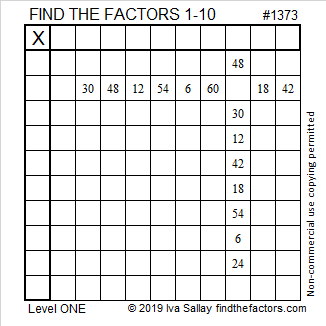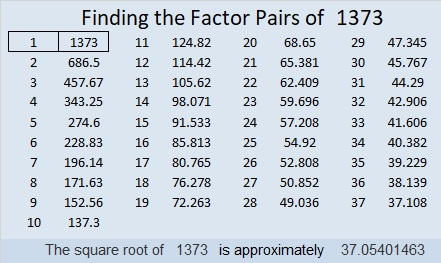# 1373 and Level 1

This puzzle is really just a multiplication table but the factors are missing and the products are not in order. You can figure out where the factors go, and then the clues will all make sense.Print the puzzles or type the solution in this excel file: 10 Factors 1373-1388

Now I’ll share some facts about the puzzle number, 1373:

• 1373 is a prime number.
• Prime factorization: 1373 is prime.
• 1373 has no exponents greater than 1 in its prime factorization, so √1373 cannot be simplified.
• The exponent in the prime factorization is 1. Adding one to that exponent we get (1 + 1) = 2. Therefore 1373 has exactly 2 factors.
• The factors of 1373 are outlined with their factor pair partners in the graphic below.How do we know that 1373 is a prime number? If 1373 were not a prime number, then it would be divisible by at least one prime number less than or equal to √1373. Since 1373 cannot be divided evenly by 2, 3, 5, 7, 11, 13, 17, 19, 23, 29, 31, or 37, we know that 1373 is a prime number.

1373 is the sum of two squares:
37² + 2² = 1373

1373 is the hypotenuse of a Pythagorean triple:
148-1365-1373 calculated from 2(37)(2), 37² – 2², 37² + 2²

Here’s another way we know that 1373 is a prime number: Since its last two digits divided by 4 leave a remainder of 1, and 37² + 2² = 1373 with 37 and 2 having no common prime factors, 1373 will be prime unless it is divisible by a prime number Pythagorean triple hypotenuse less than or equal to √1373. Since 1373 is not divisible by 5, 13, 17,  29, or 37, we know that 1373 is a prime number.